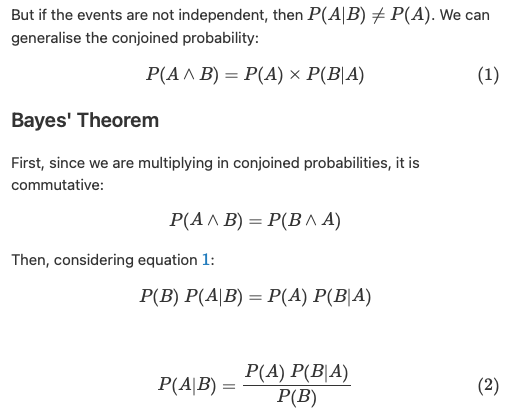# Numbering and tagging equations in MathJax

I went around in circles trying to figure out how to number and tag equations in MathJax in DT3. The MathJax Docs shows how to do automatic equation numbering but it uses \begin{equation} and \begin{equation*} to distinguish what is numbered (in the “ams” style). Pain in the derrièr to type those fences and clogs up the “Edit” pane if you have many equations. (I wouldn’t mind using them in the context of a LaTeX manuscript.)

I just wanted a way to number a few equations quickly, and tag them for reference. The $$ fence is quicker, plus I am fairly certain that hardcoding the equation numbers is not a problem going forward. This might be useful, for instance, if you are using DT3 to take notes. The effect that I wanted was this:Note the blue “1” which is a link to equation 1. The code is simple: just use \tag{} and \label{}. If you don’t need to refer to the equation you can just leave out the latter. You use \ref{} to refer to the equation inline. This is the text for the above: But if the events are not independent, then P(A|B) \neq P(A). We can generalise the conjoined probability:$$P(A \land B) = P(A) \times P(B|A) \label{cjp} \tag{1}$$### Bayes’ Theorem First, since we are multiplying in conjoined probabilities, it is commutative:$$P(A \land B) = P(B \land A)$$Then, considering equation \ref{cjp}:$$P(B)\ P(A|B) = P(A)\ P(B|A)P(A|B) = \frac{P(A)\ P(B|A)}{P(B)} \label{bayes} \tag{2}

Hope this helps you.

(Pardon any inaccuracies in the maths – I’m not a mathematician!)

1 Like

Wonderful!
Thanks for the clear explanation of your findings. I’m sure this will be helpful to many people.